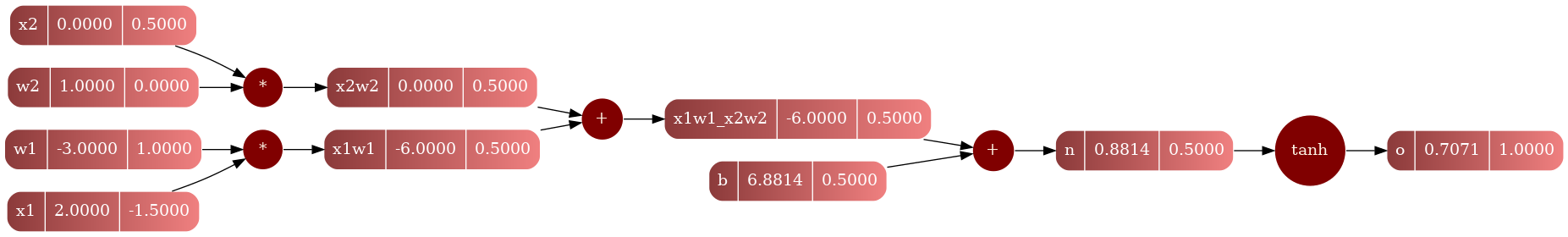Tools Used: C++, Graphviz

Topics: Deep Learning, Machine Learning

## Introduction¶

MicroGradCpp is a C++ implementation of MicroGrad, a minimalistic deep learning library. It includes an API to calculate gradients, and to train neural networks in single neuron level. It is a very simple library, and it is very easy to use. It is a very good tool to learn how neural networks work, and how they are trained.

The project is a replicate of Andrej Karpathy’s MicroGrad with a few extra features and in C++.

## Features¶

• Custom value and gradient system.
• Graphviz support for visualizing the graph.
• Fully working neural network implementation.

Here is an implementation of a single neuron:

  1 2 3 4 5 6 7 8 9 10 11 12 13 14 15 16 17 18  Value x1 = Value(2.0, "x1"); Value x2 = Value(0.0, "x2"); // weights Value w1 = Value(-3.0, "w1"); Value w2 = Value(1.0, "w2"); // bias Value b = Value(6.8813735870195432, "b"); // neuron (x1*w1 + x2*w2 + b) Value x1w1 = x1 * w1; x1w1.set_label("x1w1"); Value x2w2 = x2 * w2; x2w2.set_label("x2w2"); Value x1w1_x2w2 = x1w1 + x2w2; x1w1_x2w2.set_label("x1w1_x2w2"); Value n = x1w1_x2w2 + b; n.set_label("n"); // output w tanh Value o = n.tanh(); o.set_label("o"); o.backward(); Graph gs; gs.draw(o, "file_name"); 

Which gives the following graph: